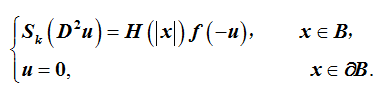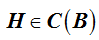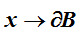# 奇异k-Hessian方程的多个径向解Many Radial Solutions of Singular k-Hessian Equations

• 全文下载: PDF(531KB)    PP.289-295   DOI: 10.12677/PM.2018.83038
• 下载量: 435  浏览量: 1,678   国家自然科学基金支持

We prove that many nontrivial radial solutions exist for the singular k-Hessian problemHereis the k-Hessian operator, and B is the unit ball in RN (N≥2) . The main interest is that the weight functionis unbounded as, and many nontrivial radial solutions to the above k-Hessian problem are derived. Our approach to show existence and multiplicity, exploits fixed point index theory.

1. 引言

K-Hession问题源自几何学，流体力学和其他应用学科。例如，当k = N时，k-Hessian问题可以表示Weingarten曲率或者是反射面形状，参见文献  。近年来，越来越多作者开始研究k-Hessian问题，并取得了很多优秀的成果，详见文献  -  。

k-Hessian方程的一般形式如下：

$\left\{\begin{array}{l}{S}_{k}\left({D}^{2}u\right)=H\left(x\right)f\left(-u\right),\text{\hspace{0.17em}}\text{\hspace{0.17em}}\text{\hspace{0.17em}}\text{\hspace{0.17em}}\text{\hspace{0.17em}}\text{\hspace{0.17em}}\text{\hspace{0.17em}}x\in \Omega ,\\ u=0,\text{\hspace{0.17em}}\text{\hspace{0.17em}}\text{\hspace{0.17em}}\text{\hspace{0.17em}}\text{\hspace{0.17em}}\text{\hspace{0.17em}}\text{\hspace{0.17em}}\text{\hspace{0.17em}}\text{\hspace{0.17em}}\text{\hspace{0.17em}}\text{\hspace{0.17em}}\text{\hspace{0.17em}}\text{\hspace{0.17em}}\text{\hspace{0.17em}}\text{\hspace{0.17em}}\text{\hspace{0.17em}}\text{\hspace{0.17em}}\text{\hspace{0.17em}}\text{\hspace{0.17em}}\text{\hspace{0.17em}}\text{\hspace{0.17em}}\text{\hspace{0.17em}}\text{\hspace{0.17em}}\text{\hspace{0.17em}}\text{\hspace{0.17em}}\text{\hspace{0.17em}}\text{\hspace{0.17em}}\text{\hspace{0.17em}}\text{\hspace{0.17em}}\text{\hspace{0.17em}}\text{\hspace{0.17em}}\text{\hspace{0.17em}}\text{\hspace{0.17em}}\text{\hspace{0.17em}}x\in \partial \Omega .\end{array}$

${S}_{k}\left({D}^{2}u\right)={P}_{k}\left(\Lambda \right)=\underset{1\le {i}_{1}<\cdots <{i}_{k}\le N}{\sum }{\lambda }_{{i}_{1}}\cdots {\lambda }_{{i}_{k}},$

$\Lambda =\left({\lambda }_{1},{\lambda }_{2},\cdots ,{\lambda }_{N}\right)$ 是Hessian矩阵 ${D}^{2}u$ 的特征值。在文献   中，Wang证明了 ${P}_{k}\left(\Lambda \right)$ 表示 $\Lambda$ 中的第k个初等对称多项式。

$\left\{\begin{array}{l}{S}_{k}\left({D}^{2}u\right)=H\left(|x|\right)f\left(-u\right),\text{\hspace{0.17em}}\text{\hspace{0.17em}}\text{\hspace{0.17em}}\text{\hspace{0.17em}}\text{\hspace{0.17em}}\text{\hspace{0.17em}}\text{\hspace{0.17em}}x\in B,\\ u=0,\text{\hspace{0.17em}}\text{\hspace{0.17em}}\text{\hspace{0.17em}}\text{\hspace{0.17em}}\text{\hspace{0.17em}}\text{\hspace{0.17em}}\text{\hspace{0.17em}}\text{\hspace{0.17em}}\text{\hspace{0.17em}}\text{\hspace{0.17em}}\text{\hspace{0.17em}}\text{\hspace{0.17em}}\text{\hspace{0.17em}}\text{\hspace{0.17em}}\text{\hspace{0.17em}}\text{\hspace{0.17em}}\text{\hspace{0.17em}}\text{\hspace{0.17em}}\text{\hspace{0.17em}}\text{\hspace{0.17em}}\text{\hspace{0.17em}}\text{\hspace{0.17em}}\text{\hspace{0.17em}}\text{\hspace{0.17em}}\text{\hspace{0.17em}}\text{\hspace{0.17em}}\text{\hspace{0.17em}}\text{\hspace{0.17em}}\text{\hspace{0.17em}}\text{\hspace{0.17em}}\text{\hspace{0.17em}}\text{\hspace{0.17em}}\text{\hspace{0.17em}}\text{\hspace{0.17em}}\text{}x\in \partial B.\end{array}$ (1.1)

1) $f\in {C}^{2}\left(\left[0,+\infty \right)\right),f\left(0\right)=0,f\left(s\right)>\text{0}\left(0\le s<+\infty \right)$

2) $-s{f}^{\prime }\left(-s\right)>kf\left(-s\right)\text{}\left(s<0\right)$

3) $-s{f}^{\prime }\left(-s\right)

2. 几个引理

${S}_{k}\left({D}^{2}u\right)={C}_{N-1}^{k-1}{t}^{1-N}\left(\frac{{t}^{N-k}}{k}{\left({u}^{\prime }\right)}^{k}\right),t=|x|,x\in {R}^{N}.$

$\left\{\begin{array}{l}{C}_{N-1}^{k-1}{t}^{1-N}{\left(\frac{{t}^{N-k}}{k}{\left(-{u}^{\prime }\right)}^{k}\right)}^{\prime }=\lambda H\left(t\right)f\left(-u\right),\text{}0 (2.1)

$\left\{\begin{array}{l}{C}_{N-1}^{k-1}{t}^{1-N}{\left(\frac{{t}^{N-k}}{k}{\left(-{v}^{\prime }\right)}^{k}\right)}^{\prime }=\lambda H\left(t\right)f\left(v\right),\text{\hspace{0.17em}}\text{\hspace{0.17em}}0 (2.2)

(H1) $f\in C\left({R}^{+},{R}^{+}\right)$ ，其中 ${R}^{+}=\left[0,+\infty \right)$

(H2) 非负函数 $H\in C\left(\left[0,1\right)\right)$${\int }_{0}^{1}H\left(t\right)\text{d}t<+\infty$ ，且其在[0,1]任意子区间上不恒为0。

1) 如果v(t)是问题(2.2)的一个解，那么 $u\left(t\right)=-v\left(t\right)$ 也是问题(2.1)在J上的一个解；

2) 如果u(t)是问题(2.1)的一个解，那么 $v\left(t\right)=-u\left(t\right)$ 也是问题(2.2)在J上的一个解。

$‖v‖=\underset{t\in J}{\mathrm{max}}|v\left(t\right)|$ 。如果 $v\in {C}^{2}\left(0,1\right)\cap {C}^{1}\left[0,1\right)$ ，且满足(2.2)式，那么称v是问题(2.2)的解。

$v\left(t\right)={{\int }_{t}^{1}\left({\int }_{0}^{\tau }k{\tau }^{k-N}{s}^{N-1}{\left({C}_{N-1}^{k-1}\right)}^{-1}H\left(s\right)fv\left(s\right)\text{d}s\right)}^{\frac{1}{k}}\text{d}\tau$ (2.3)

$\underset{t\in {J}_{\theta }}{\mathrm{min}}v\left(t\right)\ge \theta ‖v‖,$ (2.4)

$K=\left\{v\in E:v\ge 0,\underset{t\in {J}_{\theta }}{\mathrm{min}v\left(t\right)\ge \theta ‖v‖}\right\}.$

${\Omega }_{\rho }=\left\{v\in K:\underset{t\in J}{\mathrm{min}}v\left(t\right)<\gamma \rho \right\}=\left\{v\in E:\gamma ‖v‖\le \underset{t\le {J}_{\theta }}{\mathrm{min}v\left(t\right)<\gamma \rho }\right\}.$

1) ${\Omega }_{\rho }$ 是K中开集；

2) ${K}_{\gamma \rho }\subset {\Omega }_{\rho }\subset {K}_{\rho }$

3) $v\in \partial {\Omega }_{\rho }⇔\underset{t\in {J}_{\theta }}{\mathrm{min}}v\left(t\right)=\gamma \rho$

4) 如果 $v\in \partial {\Omega }_{\rho }$ ，则有 

$\left(Tv\right)\left(t\right)={\int }_{t}^{1}{\left({\int }_{0}^{\tau }k{\tau }^{k-N}{s}^{N-1}{\left({C}_{N-1}^{k-1}\right)}^{-1}H\left(s\right)f\left(v\left(s\right)\right)\text{d}s\right)}^{\frac{1}{k}}\text{d}\tau$ (2.5)

1) 如果对 $\forall x\in \partial {D}_{k},‖Ax‖\le ‖x‖$ ，则 ${i}_{k}\left(A,{D}_{k}\right)=1$

2) 如果  使得对 $\forall x\in \partial {D}_{k}$$\forall \lambda >0$$x\ne Ax+\lambda e$ 成立，则 ${i}_{k}\left(A,{D}_{k}\right)=\text{0}$

3) 设U是K中的开集且 $\stackrel{¯}{U}\in {D}_{k}$ 。如果 ${i}_{k}\left(A,{D}_{k}\right)=1$${i}_{k}\left(A,{U}_{k}\right)=\text{0}$ ，则A在 ${D}_{k}\\stackrel{¯}{{U}_{k}}$ 上有一个不动点。如果条件为 ${i}_{k}\left(A,{D}_{k}\right)=0$${i}_{k}\left(A,{U}_{k}\right)=\text{1}$ ，则结论也成立。

3. 主要结论

$d={\int }_{0}^{1}H\left(s\right)\text{d}s\text{ }\text{ },{d}_{\text{*}}={\int }_{\theta }^{1-\theta }H\left(s\right)\text{d}s,\text{ }\text{ }{f}_{\gamma \rho }^{\rho }=\mathrm{min}\left\{\frac{f\left(v\right)}{{\rho }^{k}}:v\in \left[\gamma \rho ,\rho \right]\right\};$

${f}_{0}^{\rho }=\mathrm{max}\left\{\frac{f\left(v\right)}{{\rho }^{k}}:v\in \left[0,\rho \right]\right\},\text{ }\text{ }\text{ }\text{ }{f}^{\rho }=\underset{v\to \alpha }{\mathrm{lim}}\frac{f\left(v\right)}{{v}^{k}}\left(\alpha :=\infty 或{0}^{+}\right);$

$\frac{\text{1}}{l}={\left\{dk{\left({C}_{N-1}^{k-1}\right)}^{-1}\right\}}^{\frac{1}{k}}\frac{k}{2k-N},\text{ }\text{ }\text{ }\text{ }\text{ }\frac{1}{L}={\left\{{d}_{*}k{\left({C}_{N-1}^{k-1}\right)}^{-1}\right\}}^{\frac{1}{k}}\frac{k}{2k-N}\left[1-{\left(1-\theta \right)}^{\frac{2k-N}{k}}\right].$

(H3)存在 ${\rho }_{\text{1}},{\rho }_{\text{2}},{\rho }_{3}\in \left(0,\text{+}\infty \right)$ ，且 ${\rho }_{\text{1}}<\gamma {\rho }_{2},{\rho }_{2}<{\rho }_{3}$ 使得

${f}_{\text{0}}^{{\rho }_{1}}>{l}^{k},{f}_{\gamma {\rho }_{2}}^{{\rho }_{2}}<{L}^{k},{f}_{0}^{{\rho }_{3}}>{l}^{k},$

(H4)存在 ${\rho }_{\text{1}},{\rho }_{\text{2}},{\rho }_{3}\in \left(0,\text{+}\infty \right)$ ，且 ${\rho }_{\text{1}}<{\rho }_{2}<{\rho }_{3}$ 使得

${f}_{\gamma {\rho }_{1}}^{{\rho }_{1}}>{L}^{k},{f}_{0}^{{\rho }_{2}}<{l}^{k},{f}_{\gamma {\rho }_{3}}^{{\rho }_{3}}>{L}^{k},$

1) 问题(2.2)至少有两个正解 ${v}_{1},{v}_{2}$ 满足 ${v}_{1}\in {\Omega }_{{\rho }_{2}}\\stackrel{¯}{{K}_{{\rho }_{1}}},{v}_{2}\in {K}_{{\rho }_{3}}\\stackrel{¯}{{\Omega }_{{\rho }_{2}}}$

2) 问题(1.1)至少有两个非平凡径向解 ${u}_{1},{u}_{2}$ 满足 ${u}_{1}=-{v}_{1},{u}_{2}=-{v}_{2}$

$\begin{array}{c}\left(Tv\right)\left(t\right)={\int }_{t}^{1}{\left({\int }_{0}^{\tau }k{\tau }^{k-N}{s}^{N-1}{\left({C}_{N-1}^{k-1}\right)}^{-1}H\left(s\right)f\left(v\left(s\right)\right)\text{d}s\right)}^{\frac{1}{k}}\text{d}\tau \\ \le {\int }_{0}^{1}{\left({\int }_{0}^{1}k{\tau }^{k-N}{s}^{N-1}{\left({C}_{N-1}^{k-1}\right)}^{-1}H\left(s\right)f\left(v\left(s\right)\right)\text{d}s\right)}^{\frac{1}{k}}\text{d}\tau <{\int }_{0}^{1}{\left({\int }_{0}^{1}k{\tau }^{k-N}{s}^{N-1}{\left({C}_{N-1}^{k-1}\right)}^{-1}H\left(s\right){l}^{k}{\rho }_{1}^{k}\text{d}s\right)}^{\frac{1}{k}}\text{d}\tau \\ ={\left(k{\left({C}_{N-1}^{k-1}\right)}^{-1}\right)}^{\frac{1}{k}}l{\rho }_{1}{{\int }_{0}^{1}\tau }^{\frac{k-N}{k}}\text{d}\tau {\left({\int }_{0}^{1}{s}^{N-1}H\left(s\right)\text{d}s\right)}^{\frac{1}{k}}\le {\left(k{\left({C}_{N-1}^{k-1}\right)}^{-1}\right)}^{\frac{1}{k}}l{\rho }_{1}{{\int }_{0}^{1}\tau }^{\frac{k-N}{k}}\text{d}\tau {\left({\int }_{0}^{1}H\left(s\right)\text{d}s\right)}^{\frac{1}{k}}\\ ={\left(dk{\left({C}_{N-1}^{k-1}\right)}^{-1}\right)}^{\frac{1}{k}}l{\rho }_{1}\frac{k}{2k-N},\end{array}$

$e\left(t\right)\equiv 1$ ，则 $e\in \partial {K}_{1}$ 。事实上，有

$v\ne Tv+\lambda e,v\in \partial {\Omega }_{{\rho }_{2}},\lambda >0,$

${v}_{0}=T{v}_{0}+{\lambda }_{0}e.$ (3.1)

$\begin{array}{c}{v}_{0}=T{v}_{0}+{\lambda }_{0}e\ge \gamma ‖T{v}_{0}‖+{\lambda }_{0}e\\ \ge \gamma {\int }_{1-\theta }^{\text{1}}{\left({\int }_{\theta }^{1-\theta }k{\tau }^{k-N}{s}^{N-1}{\left({C}_{N-1}^{k-1}\right)}^{-1}H\left(s\right)f\left({v}_{0}\left(s\right)\right)\text{d}s\right)}^{\frac{1}{k}}\text{d}\tau +{\lambda }_{0}\\ >\gamma L{\rho }_{2}{\int }_{1-\theta }^{\text{1}}{\left({\int }_{\theta }^{1-\theta }k{\tau }^{k-N}{s}^{N-1}{\left({C}_{N-1}^{k-1}\right)}^{-1}H\left(s\right)\text{d}s\right)}^{\frac{1}{k}}\text{d}\tau +{\lambda }_{0}\text{ }\\ =\gamma L{\rho }_{2}{\left(k{\left({C}_{N-1}^{k-1}\right)}^{-1}\right)}^{\frac{1}{k}}{\int }_{1-\theta }^{1}{\tau }^{\frac{k-N}{k}}\text{d}\tau {\left({\int }_{\theta }^{1-\theta }{s}^{N-1}H\left(s\right)\text{d}s\right)}^{\frac{1}{k}}+{\lambda }_{0}\end{array}$

$\begin{array}{c}\ge \gamma L{\rho }_{2}{\left(k{\left({C}_{N-1}^{k-1}\right)}^{-1}\right)}^{\frac{1}{k}}{\int }_{1-\theta }^{1}{\tau }^{\frac{k-N}{k}}\text{d}\tau {\left({\int }_{\theta }^{1-\theta }H\left(s\right)\text{d}s\right)}^{\frac{1}{k}}+{\lambda }_{0}\\ =\gamma L{\rho }_{2}{\left(k{\left({C}_{N-1}^{k-1}\right)}^{-1}\right)}^{\frac{1}{k}}\frac{k}{2k-N}\left[1-{\left(1-\theta \right)}^{\frac{2k-N}{k}}\right]+{\lambda }_{0}\\ =\gamma {\rho }_{2}+{\lambda }_{0},\end{array}$

1) 如果存在 ${\left\{{\rho }_{i}\right\}}_{i=1}^{2{m}_{0}}\subset \left(0,\text{+}\infty \right),$ 满足 ${\rho }_{\text{1}}<\gamma {\rho }_{2}<{\rho }_{2}<{\rho }_{3}<\gamma {\rho }_{4}<\cdots <{\rho }_{2{m}_{0}}$ ，使得

${f}_{0}^{{\rho }_{2m-1}}<{l}^{k},{f}_{\gamma {\rho }_{2m}}^{{\rho }_{2m}}>{L}^{k},m=1,2,\cdots ,{m}_{0},$

i) 问题(2.2)在K中至少有2m0个正解，

ii) 问题(1.1)至少有2m0个非负径向解。

2) 如果存在 ${\left\{{\rho }_{i}\right\}}_{i=1}^{2{m}_{0}}\subset \left(0,\infty \right),$ 满足 ${\rho }_{\text{1}}<{\rho }_{\text{2}}$${\rho }_{2}<\gamma {\rho }_{3}<{\rho }_{3}<{\rho }_{4}<\gamma {\rho }_{5}<\cdots <{\rho }_{2{m}_{0}+2}$ ，使得

${f}_{\gamma {\rho }_{2m-1}}^{{\rho }_{2m-1}}<{L}^{k},{f}_{0}^{{\rho }_{mk}}>{l}^{k},m=1,2,\cdots ,{m}_{0},$

i) 问题(2.2)在K中至少有2m0－1个正解，

ii) 问题(1.1)至少有2m0－1个非负径向解。

  Trudinger, N. and Wang, X. (2008) The Monge-Ampère Equation and Its Geometric Applications. Handbook of Geometric Analysis, 1, 467-524. http://pdfs.semanticscholar.org/3e98/fded69b4c929d882393a609831df67a48e92.pdf  Brandolini, B. (2013) On the Symmetry of Solutions to a k-Hessian Type Equation. Advanced Nonlinear Studies, 13, 487-493. https://doi.org/10.1515/ans-2013-0213  Della Pietra, F. and Gavitone, N. (2014) Upper Bounds for the Eigenvalues of Hessian Equations. Annali di Matematica Pura ed Applicata, 193, 923-938. https://doi.org/10.1007/s10231-012-0307-5  Gavitone, N. (2009) Isoperimetric Estimates for Eigenfunctions of Hessian Operators. Ricerche di Matematica, 58, 163-183. https://doi.org/10.1007/s11587-009-0058-9  Zhang, X. and Feng, M. (2018) Boundary Blow-Up Solutions to the k-Hessian Equation with Singular Weights. Nonlinear Analysis, 167, 51-66. https://doi.org/10.1016/j.na.2017.11.001  Sánchez, J. and Vergara, V. (2016) Bounded Solutions of a k-Hessian Equation in a Ball. Journal of Differential Equations, 261, 797-820. https://doi.org/10.1016/j.jde.2016.03.021  Nakamori, S. and Takimoto, K. (2015) A Ernstein Type Theorem for Parabolic k-Hessian Equations. Nonlinear Analysis, 117, 211-220. https://doi.org/10.1016/j.na.2015.01.010  Wang, Q. and Xu, C.-J. (2014) C1;1 Solution of the Dirichlet Problem for Dege-nerate k-Hessian Equations. Nonlinear Analysis, 104, 133-146. https://doi.org/10.1016/j.na.2014.03.016  Huang, Y. (2010) Boundary Asymptotical Behavior of Large Solutions to Hessian Equations. Pacific Journal of Mathematics, 244, 85-98. https://doi.org/10.2140/pjm.2010.244.85  Dai, L. and Bao, J. (2011) On Uniqueness and Existence of Viscosity Solutions to Hessian Equations in Exterior Domains. Frontiers of Mathematics in China, 6, 221-230. https://doi.org/10.1007/s11464-011-0109-x  Wang, C. and Bao, J. (2013) Necessary and Sufficient Conditions on Existence and Convexity of Solutions for Dirichlet Problems of Hessian Equations on Exterior Domains. Proceedings of the American Mathematical Society, 141, 1289-1296. https://doi.org/10.1090/S0002-9939-2012-11738-1  Urbas, J.I.E. (1990) On the Existence of Nonclassical Solutions for Two Classes of Fully Nonlinear Elliptic Equations. Indiana University Mathematics Journal, 39, 355-382. https://doi.org/10.1512/iumj.1990.39.39020  Wang, X.-J. (1994) A Class of Fully Nonlinear Elliptic Equations and Related Functionals. Indiana University Mathematics Journal, 43, 25-54. https://doi.org/10.1512/iumj.1994.43.43002  Wang, X.-J. (2009) The k-Hessian Equation, in: Geometric Analysis and PDEs. Lecture Notes in Mathematics, 1977, 177-252. https://doi.org/10.1007/978-3-642-01674-5_5  Clément, P., De Fi-gueiredo, D.G. and Mitidieri, E. (1996) Quasilinear Elliptic Equations with Critical Exponents. Topological Methods in Nonlinear Analysis, 7, 133-170. https://doi.org/10.12775/TMNA.1996.006  Wang, F. and An, Y. (2012) Triple Nontrivial Radial Convex Solutions of Systems of Monge-Ampère Equations. Applied Mathematics Letters, 25, 88-92. https://doi.org/10.1016/j.aml.2011.07.016  Wang, H. (2006) Convex Solutions of Boundary Value Problems. Journal of Mathematical Analysis and Applications, 318, 246-252. https://doi.org/10.1016/j.jmaa.2005.05.067  Han, X., Ma, R. and Dai, G. (2015) Eigenvalue, Bifurcation and Convex Solutions for Monge-Ampère Equations. Topological Methods in Nonlinear Analysis, 45, 135-164. https://doi.org/10.12775/TMNA.2015.041  Sánchez, J. and Vergara, V. (2017) Bounded Solutions of a k-Hessian Equation Involving a Weighted Nonlinear Source. Journal of Differential Equations, 263, 687-708. https://doi.org/10.1016/j.jde.2017.02.047  Jacobsen, J. (1999) Global Bifurcation Problems Associated with k-Hessian Op-erators. Topological Methods in Nonlinear Analysis, 14, 81-130. https://doi.org/10.1016/j.jde.2017.02.047  Escudero, C. and Torres, P.J. (2015) Existence of Radial Solutions to Biharmonic K-Hessian Equations. Journal of Differential Equations, 259, 2732-2761. https://doi.org/10.1016/j.jde.2015.04.001  Zhang, Z. and Zhou, Y. (2015) Existence of Entire Positive k-Convex Radial Solutions to Hessian Equations and Systems with Weights. Applied Mathematics Letters, 50, 48-55. https://doi.org/10.1016/j.aml.2015.05.018  Wei, W. (2016) Uniqueness Theorems for Negative Radial Solutions of k-Hessian Equations in a Ball. Journal of Differential Equations, 261, 3756-3771. https://doi.org/10.1016/j.jde.2016.06.004  Lan, K. (2001) Multive Positive Solutions of Semilinear Differential Equations with Singularities. Journal of the London Mathematical Society, 63, 690-704. https://doi.org/10.1112/S002461070100206X